# Python-Matplotlib 可视化（8），毕业工作 5 年被裁

• 2022 年 3 月 21 日
• 本文字数：2193 字

阅读完需：约 7 分钟

body {

background: white url(background.jpg);

}

</style>

<body>

<img src='sinc.png' width='800 height='600'></img>

</body>

</html>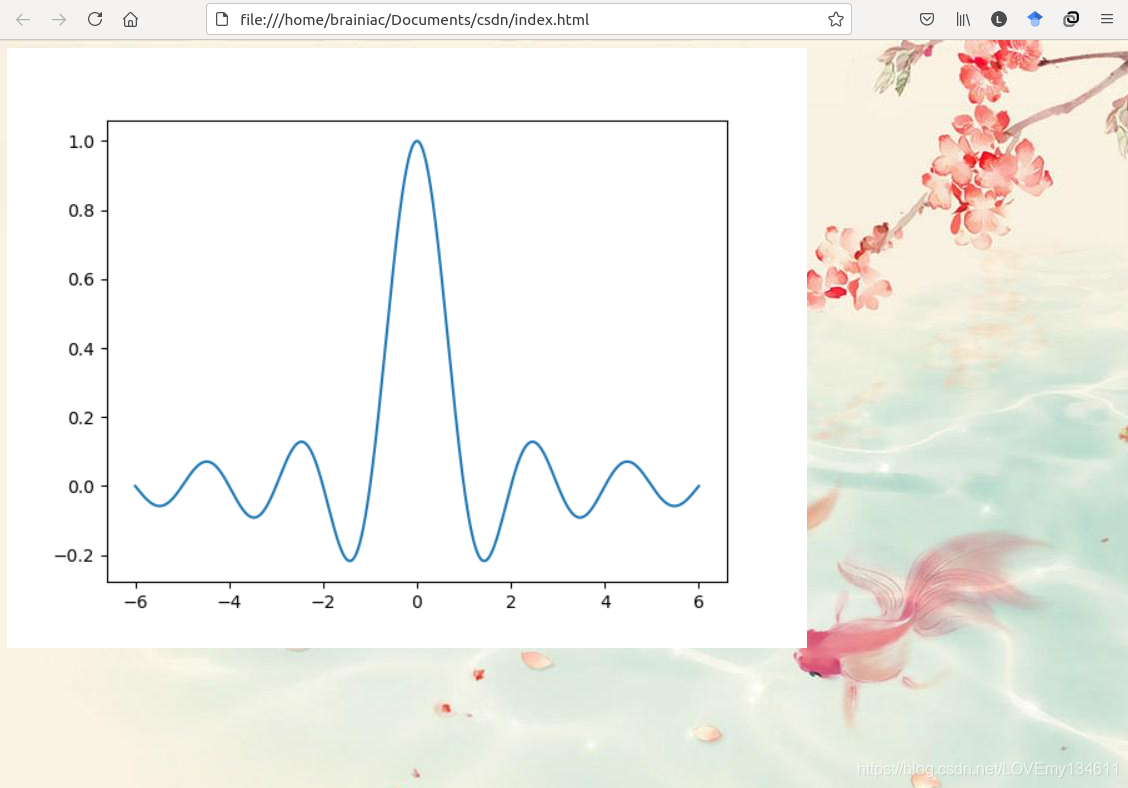import numpy as np

import matplotlib.pyplot as plt

name_list = ('Omar', 'Serguey', 'Max', 'Zhou', 'Abidin')

value_list = np.random.randint(99, size=len(name_list))

pos_list = np.arange(len(name_list))

plt.bar(pos_list, value_list, alpha = .75, color = 'c', align = 'center')

plt.xticks(pos_list, name_list)

plt.savefig('bar.png', transparent = True)

<html>

<style>

body {

background: white url(background.jpg);

}

</style>

<body>

<img src='bar.png' width='800 height='600'></img>

</body>

</html>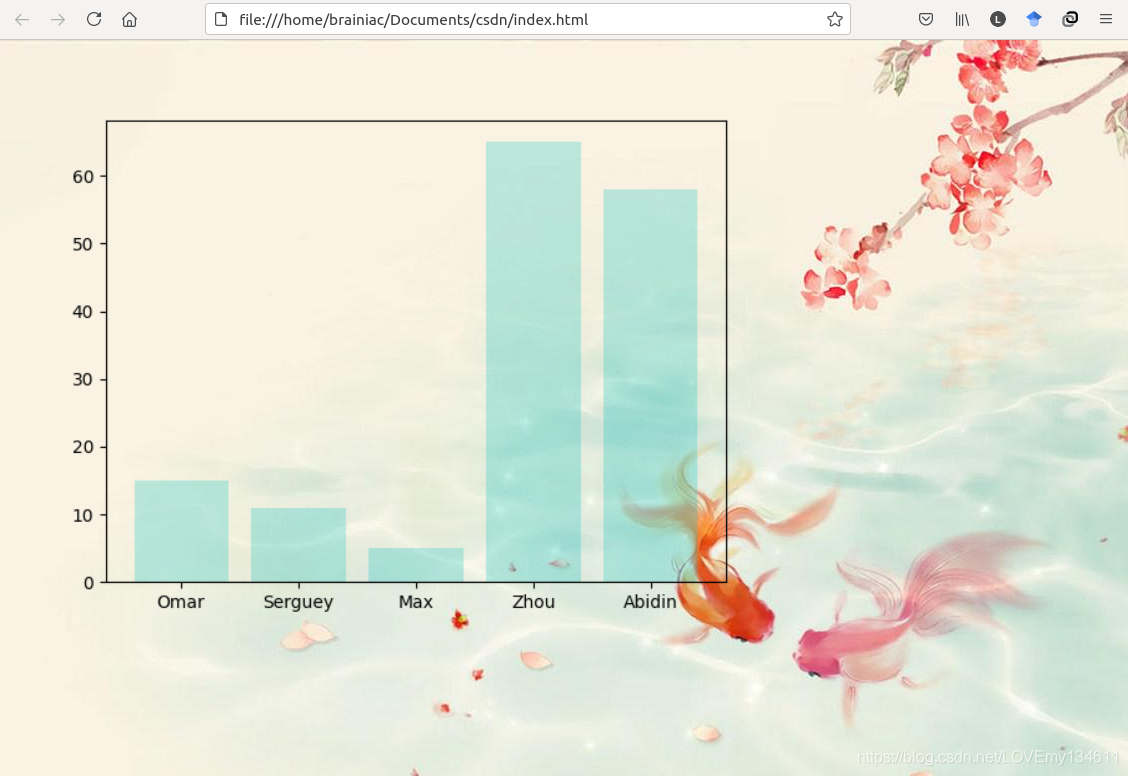[](

)控制输出分辨率

plt.savefig()函数提供可选参数dpi控制输出分辨率：

import numpy as np

import matplotlib.pyplot as plt

name_list = ('Omar', 'Serguey', 'Max', 'Zhou', 'Abidin')

value_list = np.random.randint(99, size=len(name_list))

pos_list = np.arange(len(name_list))

plt.bar(pos_list, value_list, alpha = .25, color = 'c', align = 'center')

plt.xticks(pos_list, name_list)

plt.savefig('bar_1.png', dpi = 300)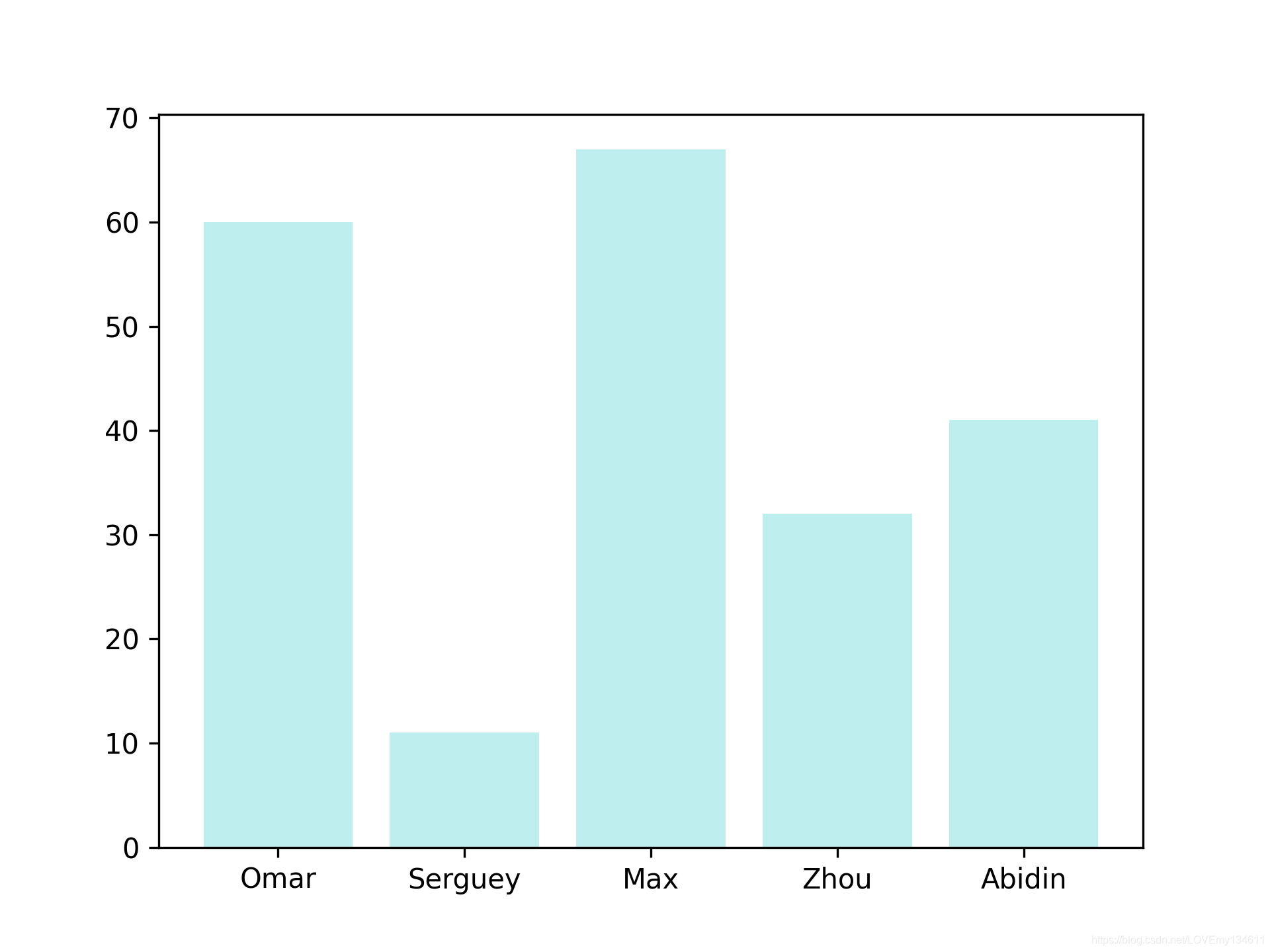Tips：savefig()函数的可选参数dpi控制以DPI(每英寸点数)表示的图片分辨率。默认情况下，Matplotlib将输出8 x 6空间单位的图形，即4/3的纵横比。在matplotlib中，1个空间单位等于100像素。因此，默认情况下，Matplotlib提供800 x 600像素的图片文件。如果我们使用dpi=300，图片大小为8*300 x 6*300，即2400 x 1800像素。

import numpy as np

import matplotlib.pyplot as plt

theta = np.linspace(0, 2 * np.pi, 8)

points = np.vstack((np.cos(theta), np.sin(theta))).transpose()

plt.figure(figsize=(4., 4.))

plt.gca().add_patch(plt.Polygon(points, color = 'c'))

plt.grid(True)

plt.axis('scaled')

plt.savefig('polygon.png', dpi = 128)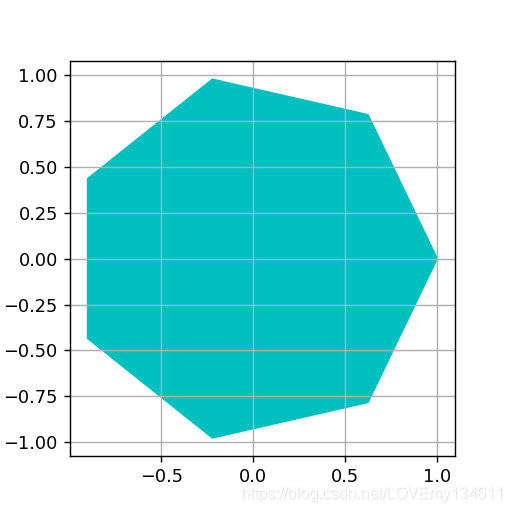import numpy as np

import matplotlib.pyplot as plt

theta = np.linspace(0, 2 * np.pi, 8)

points = np.vstack((np.cos(theta), np.sin(theta))).transpose()

plt.figure(figsize=(8., 8.))

plt.gca().add_patch(plt.Polygon(points, color = 'c'))

plt.grid(True)

plt.axis('scaled')

plt.savefig('polygon_1.png', dpi = 64)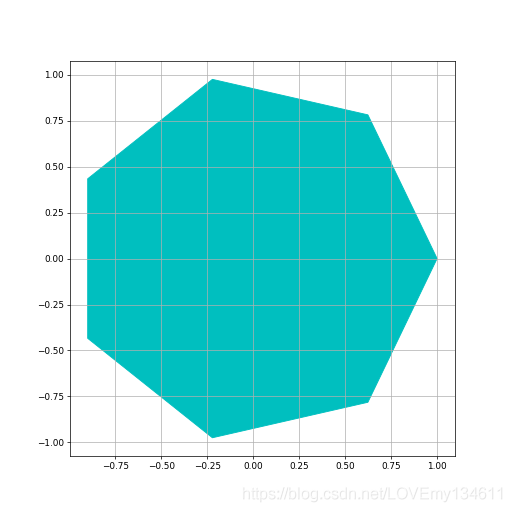Tips：图中注释更小，网格线更细，这是由于注释和线条的粗细都有自己的默认值，它们以空间单位表示。因此，将输出分辨率除以2将使注释变小两倍。

[](

)生成 PDF 或 SVG 文档

import numpy as np

from matplotlib import pyplot as plt

x = np.linspace(-10, 10, 1024)

y = np.sinc(x)

plt.plot(x, y)

plt.savefig('sinc.pdf')

Tips：调用plt.savefig()时，其中通过传递文件名来指定文件是PNG、PDF还是SVG，Matplotlib将通过文件名的扩展名来推断文件类型。

[](

)系列链接

[Python-Matplotlib 可视化（1）——一文详解常见统计图的绘制](

)

[Python-Matplotlib 可视化（2）——自定义颜色绘制精美统计图](

)

[Python-Matplotlib 可视化（3）——自定义样式绘制精美统计图](

)

[Python-Matplotlib 可视化（4）——添加注释让统计图通俗易懂](

)

[Python-Matplotlib 可视化（5）——添加自定义形状绘制复杂图形](

)

[Python-Matplotlib 可视化（6）——自定义坐标轴让统计图清晰易懂](

)

[Python-Matplotlib 可视化（7）——多方面自定义统计图绘制](

)

[Python-Matplotlib 可视化（9）——精通更多实用图形的绘制](

)

[Python-Matplotlib 可视化（10）——一文详解 3D 统计图的绘制](

)

### 最后

?? 硬核资料：关注即可领取 PPT 模板、简历模板、行业经典书籍 PDF。

?? 技术互助：技术群大佬指点迷津，你的问题可能不是问题，求资源在群里喊一声。

?? 面试题库：由技术群里的小伙伴们共同投稿，热乎的大厂面试真题，持续更新中。

?? 知识体系：含编程语言、算法、大数据生### 程序媛可鸥

Python编程资料加Q群免费领取：419829237 2022.03.14 加入

## 评论